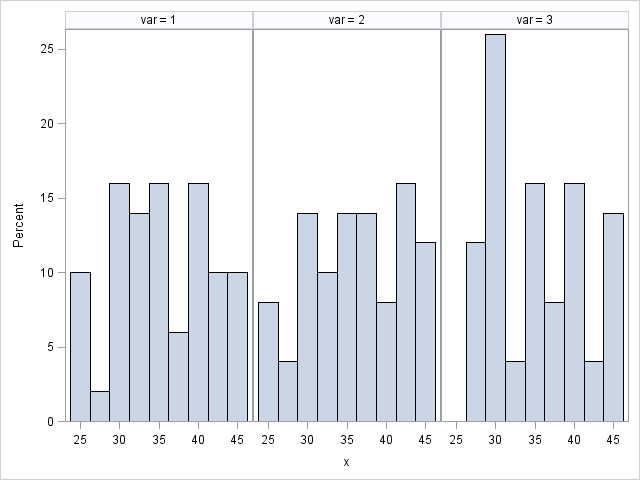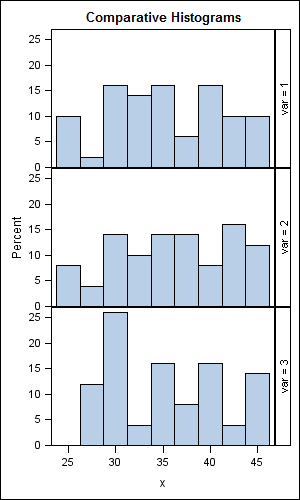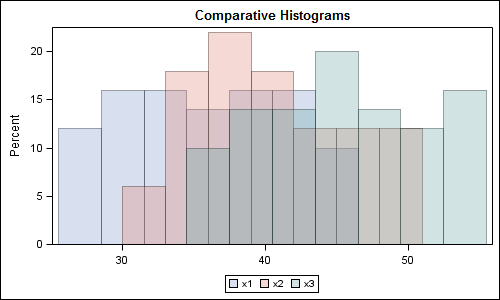## Three variables in a single graph

I want to show the distribution of three variables Preop__Hct,Postop__Hct_Day_1,Postop__Hct_Day_2 in one graph. i'm using the following code.

in the output, the values on x-axis and Y-axis are displayed as the values of these three varaibles are super imposed on one another.

what to do so that i can clearly show the distribution of three variables in one single graph.

title 'Preop,DAY1,DAY2 HCT';

goptions nodisplay;
axis1 order=(0 to 100 by 5) value=(h=2) label=(h=2);
proc univariate data=research noprint;
var Preop__Hct;
histogram Preop__Hct / normal(noprint color=red)
nobars
name='PREOPHCT'
midpoints=20 to 45
vscale=count
vaxis=axis1
height=2;
inset normal(mu sigma) / pos=nw header='PreopHCT' height=2;
run;
quit;
proc univariate data=research noprint;
var Postop__Hct_Day_1;
histogram Postop__Hct_Day_1 / normal(noprint color=blue l=20)
nobars
name='DAY1HCT'
midpoints=20 to 45
vscale=count
vaxis=axis1
height=2;
inset normal(mu sigma) / pos=ne header='DAY1HCT' height=2;
run;
quit;
proc univariate data=research noprint;
var Postop__Hct_Day_2;
histogram Postop__Hct_Day_2 / normal(noprint color=GREEN l=20)
nobars
name='DAY2HCT'
midpoints=20 to 45
vscale=count
vaxis=axis1
height=2;
inset normal(mu sigma) / pos=n header='DAY2HCT' height=2;
run;
quit;
goptions display;

/* Replay the graphs into the same template */
proc greplay igout=work.gseg nofs tc=sashelp.templt template=whole;
treplay 1:PreopHCT 1:DAY1HCT 1:DAY2HCT;

quit;
run;

4 REPLIES 4MikeZdeb
Rhodochrosite | Level 12

## Re: Three variables in a single graph

hi ...  if you want to stick to the above approach, try a different template (though not sure if the graphics will look distorted)

there are four, sas-supplied templates in the sashelp.templt library you can try:  v3, v3s, h3, h3s

proc greplay igout=work.gseg nofs tc=sashelp.templt template=v3;

treplay 1:PreopHCT 2:DAY1HCT 3:DAY2HCT;

quit;

## Re: Three variables in a single graph

I tried. Can we still plot graphs on one set of x and y axis values?

say for instance x axis will values 20 to 45 and y axis will have values 0 to 100 and we would want to see how the three varaibles are distributed over these x and y axes.MikeZdeb
Rhodochrosite | Level 12

## Re: Three variables in a single graph

hi ... I have not used the SG procs much, but here's an example that I think does something like you want ...

data test;

do var = 1 to 3;

do j = 1 to 50;

x = ceil(20*ranuni(999)) + 25;

y = ceil(100*normal(999));

output;

end;

end;

drop j;

run;

ods html path='z:\' file='test.html' (url=none);

proc sgpanel data=test noautolegend;

panelby var / layout=columnlattice;

histogram x;

run;

ods html close;

it produced the attachedJay54
Meteorite | Level 14

## Re: Three variables in a single graph

Using Mike's code, you can make a RowLattice to compare the distributions, or overlay each variable on the same plot:

data test;
do var = 1 to 3;
do j = 1 to 50;
x = ceil(20*ranuni(999)) + 25;
output;
end;
end;
drop j;
run;

ods html close;
ods graphics / reset width=3in height=5in imagename='MultiHist_RowLattice';
title 'Comparative Histograms';
proc sgpanel data=test noautolegend;
panelby var / layout=rowlattice;
histogram x;
rowaxis offsetmin=0;
run;

data test2;
do j = 1 to 50;
x1 = ceil(20*ranuni(999)) + 25;
x2 = ceil(20*ranuni(999)) + 30;
x3 = ceil(20*ranuni(999)) + 35;
output;
end;
drop j;
run;

ods graphics / reset width=5in height=3in imagename='MultiHist_Overlay';

title 'Comparative Histograms';

proc sgplot data=test2;

histogram x1 / transparency=0.7;

histogram x2 / transparency=0.7;

histogram x3 / transparency=0.7;

yaxis offsetmin=0;

xaxis display=(nolabel);

run;

This second version works better with SAS9.3 where you can control the bins.  Or, with SAS 9.2, you can use GTL to do that.

here is a related article:  Comparative Density Plots

Images attached.Discussion stats
• 4 replies
• 1211 views
• 0 likes
• 3 in conversation